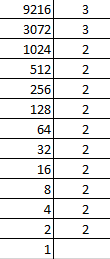Courses
Courses for Kids
Free study material
Free LIVE classes
MoreLIVE
Join Vedantu’s FREE Mastercalss

# Find the square root of the 9216 by the prime factorisation method.${\text{A}}{\text{. 96}} \\ {\text{B}}{\text{. 86}} \\ {\text{C}}{\text{. 106}} \\ {\text{D}}{\text{. 76}} \\$Verified
262.1k+ views
Hint: In this question we have to find the square root of a number using prime factorisation. In order to solve this question, we will prime factorise the given number and then take its square root. This will eventually get us the answer.

We have been given the number 9216.

Now, we have to do its prime factorisation.So, we get, $9216 = {2^{10}} \times {3^2}$

So, the square root of 9216 will be,

$\sqrt {9216} = \sqrt {{2^{10}} \times {3^2}}$

$\Rightarrow \sqrt {9216} = {2^5} \times 3 = 96$

So, the square root of the 9216 is 96.

Hence the correct option is A.

Note: Whenever we face such types of problems the value point to remember is that we need to have a good grasp over number theory. We must also do prime factorisation cautiously as it is very prone to error. This helps in getting us the required expressions and gets us on the right track to reach the answer.
Last updated date: 21st Sep 2023
Total views: 262.1k
Views today: 2.62k